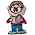## Thursday, January 14, 2010

### Letter Equations Seven Through Nine

7 = D of the W.
7 = W of the (A) W.
7 = S of the W.
7 = H of R.
7 = C of the R.
7 = D S.
7 = P of W.
7 = B for S B.
7 = S on a F P P.
7 = Y of B L for B a M.

8 = C on a C.
8 = B in a B.
8 = L on a S.
8 = T on an O.
8 = D of H.
8 = S on a S S.
8 = N in an O.
8 = U in the I L.
8 = P in a G.
8 = F in a M.
8 = P in the S S (since 2006).

9 = P in the S S (1930-2006).
9 = J on the S C.
9 = L of a C.

1.Missed a few, but here's what I got:
7 Days of the Week
7 Wonders of the (Ancient) World
7 S of the W...???
7 Hills of Rome
7 Colours of the Rainbow
7 Pillars of Wisdom
7 Brides for 7 Brothers
7 S on a FPP...???
7 Years of Bad Luck for Breaking a Mirror

8 Corners on a Cube
8 Bits in a Byte
8 Legs on a Spider
8 Tentacles on an Octopus
8 Days of Hanukkah
8 Sides on a Stop Sign
8 Notes in an Octave
8 U in the IL...???
8 Pints in a Gallon
8 Furlongs in a Mile
8 Planets in the Solar System (since 2006)

9 Planets in the Solar System (1930 - 2006)
9 Justices of the Supreme Court
9 Lives of a Cat

2.7 = Days of the Week.
7 = Wonders of the (Ancient) World.
7 = Seas of the World.
7 = Hills of Rom.
7 = Colors of the Rainbow.
7 = Pillars of Wisdom.
7 = Brides for Seven Brothers.
7 = Sides on a Fifty Pence Piece.
7 = Years of Bad Luck for Breaking a Mirror.

8 = Corners on a Cube.
8 = Bits in a Byte.
8 = Legs on a Spider.
8 = Tentacles on an Octupus.
8 = Days of Hannukah.
8 = Sides on a Stop Sign.
8 = Notes in an Octave.
8 = Universities in the Ivy League.
8 = Pints in a Gallon.
8 = Furlongs in a Mile.
8 = Planets in the Solar System (since 2006).

9 = Planets in the Solar System (1930-2006).
9 = Justices on the Supreme Court.
9 = Lives of a Cat.

Leave your answer or, if you want to post a question of your own, send me an e-mail. Look in the about section to find my e-mail address. If it's new, I'll post it soon.

Please don't leave spam or 'Awesome blog, come visit mine' messages. I'll delete them soon after.

Enter your Email and join hundreds of others who get their Question of the Day sent right to their mailbox

The Lamplight Manor Puzz 3-D